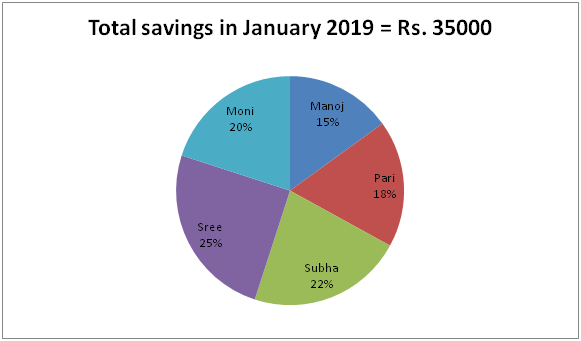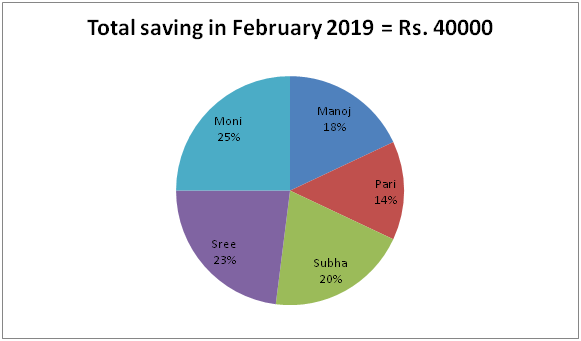# LIC AAO/SBI PO Prelims Quantitative Aptitude Questions 2019 (Day-15)

Dear Aspirants, Our IBPS Guide team is providing new series of Quantitative Aptitude Questions for LIC AAO/SBI PO 2019 so the aspirants can practice it on a daily basis. These questions are framed by our skilled experts after understanding your needs thoroughly. Aspirants can practice these new series questions daily to familiarize with the exact exam pattern and make your preparation effective.

[WpProQuiz 5742]

### Click Here for SBI PO Pre 2019 High-Quality Mocks Exactly on SBI Standard

Directions (1 – 5): What approximate value should come in place of (?) in the following questions?

1) (24.12)2 ÷ (4.05)2 × 120.11 ÷ 10.13 =? – 551.9 ÷ 3.98

a) 480

b) 1250

c) 570

d) 760

e) 1020

2) 13 (4/5) + 8 (1/10) – 11 (2/5) + 16 (3/10) =? – 3 (4/5)

a) 30

b) 20

c) 25

d) 32

e) 28

3) 9 1/7 % of 8398 + 25 1/3 % of 2699 = ? – 58 % of 1097

a) 1960

b) 1730

c) 1040

d) 2070

e) 2850

4) 2204 ÷ 63 % of 697 + 28 % of 1248 =?

a) 460

b) 355

c) 510

d) 275

e) 380

5) (7/15) of 6344.89 + 108 % of 1246 =? + 2764.7

a) 1546

b) 1972

c) 1784

d) 1650

e) 2138

Directions (6 – 10): Study the following information carefully and answer the given questions:

The following pie chart -1 shows the percentage distribution of savings of 5 different friends in January 2019 and pie chart – 2 shows the percentage distribution of savings of 5 friends in February 2019.6) The savings of Manoj and Pari together in January 2019 is what percentage more/less than the savings of Pari and Subha together in February 2019?

a) 15 % more

b) 25 % less

c) 15 % less

d) 25 % more

e) 20 % more

7) Find the difference between the average savings of Sree and Moni together in January 2019 to that of the average savings of Moni and Manoj together in February 2019?

a) 725

b) Rs. 900

c) Rs. 850

d) Rs. 675

e) None of these

8) Find the ratio between the savings of Manoj and Subha together in January 2019 to that of the savings of Pari and Moni together in February 2019?

a) 315: 223

b) 447: 335

c) 15: 7

d) 259: 312

e) None of these

9) Total savings of Moni in January 2019 is what percentage of total savings of Pari in February 2019?

a) 150 %

b) 125 %

c) 140 %

d) 105 %

e) None of these

10) Find the total savings of Manoj and Subha in January and February together?

a) Rs. 26800

b) Rs. 24550

c) 28150

d) Rs. 32500

e) None of these

Direction (1-5) :

242 ÷ 42 × 120 ÷ 10 = x – (552/4)

[(24*24)/(4*4)]*(120/10) = x – (552/4)

432 + 138 = x

= > x = 570

13 (4/5) + 8 (1/10) – 11 (3/5) + 16 (3/10) = x – 3 (4/5)

14 + 8 – 12 + 16 + 4 = x

= > x = 30

9 % of 8400 + 25 % of 2700 + 58 % of 1100 = x

X = (9/100)*8400 + (25/100)*2700 + (58/100)*1100

X = 756 + 675 + 638 = 2069 = 2070

2205 ÷ 63 % of 700 + 28 % of 1250 = x

X = 2205 ÷ [(63/100)*700] + (28/100)*1250

X = 2205/441 + 350

X = 5 + 350 = 355

(7/15)*6345 + (108/100)*1250 = x + 2765

2961 + 1350 – 2765 = x

X = 1546

Direction (6-10) :

The savings of Manoj and Pari together in January 2019

= > (33/100)*35000 = 11550

The savings of Pari and Subha together in February 2019

= > (34/100)*40000 = 13600

Required % = [(13600 – 11550)/13600]*100 = 15 % less

The average savings of Sree and Moni together in January 2019

= > (45/100)*35000*(1/2) = 7875

The average savings of Moni and Manoj together in February 2019

= > (43/100)*40000*(1/2) = 8600

Required difference = 8600 – 7875 = Rs. 725

The savings of Manoj and Subha together in January 2019

= > (37/100)*35000

The savings of Pari and Moni together in February 2019

= > (39/100)*40000

Required ratio = [(37/100)*35000]: [(39/100)*40000] = 259: 312

Total savings of Moni in January 2019

= > (20/100)*35000 = 7000

Total savings of Pari in February 2019

= > (14/100)*40000 = 5600

Required % = (7000/5600)*100 = 125 %

The total savings of Manoj and Subha in January

= > (37/100)*35000 = Rs. 12950

The total savings of Manoj and Subha in February

= > (38/100)*40000 = Rs. 15200

Required sum = 12950 + 15200 = Rs. 28150